# Power of a statistical test

(diff) ← Older revision | Latest revision (diff) | Newer revision → (diff)
The probability with which a statistical test for testing a simple hypothesis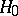against a simple hypothesisrejectswhen in fact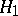is true. In the case when the hypothesis, competing within the test, is compound (itself may be either simple or compound, which is written symbolically::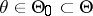,:), the power of the statistical test foragainstis defined as the restriction of the power function,, of this test to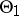.
In addition, this definition has been broadly generalized to the following: The power of a statistical test for testing:against a compound alternative: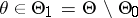is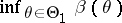, where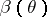is the power function of the test (see Power function of a test).